# Math for the Master(y)

Silver Volume now available in individual spiral-bound units OR original hardcover set while supplies last!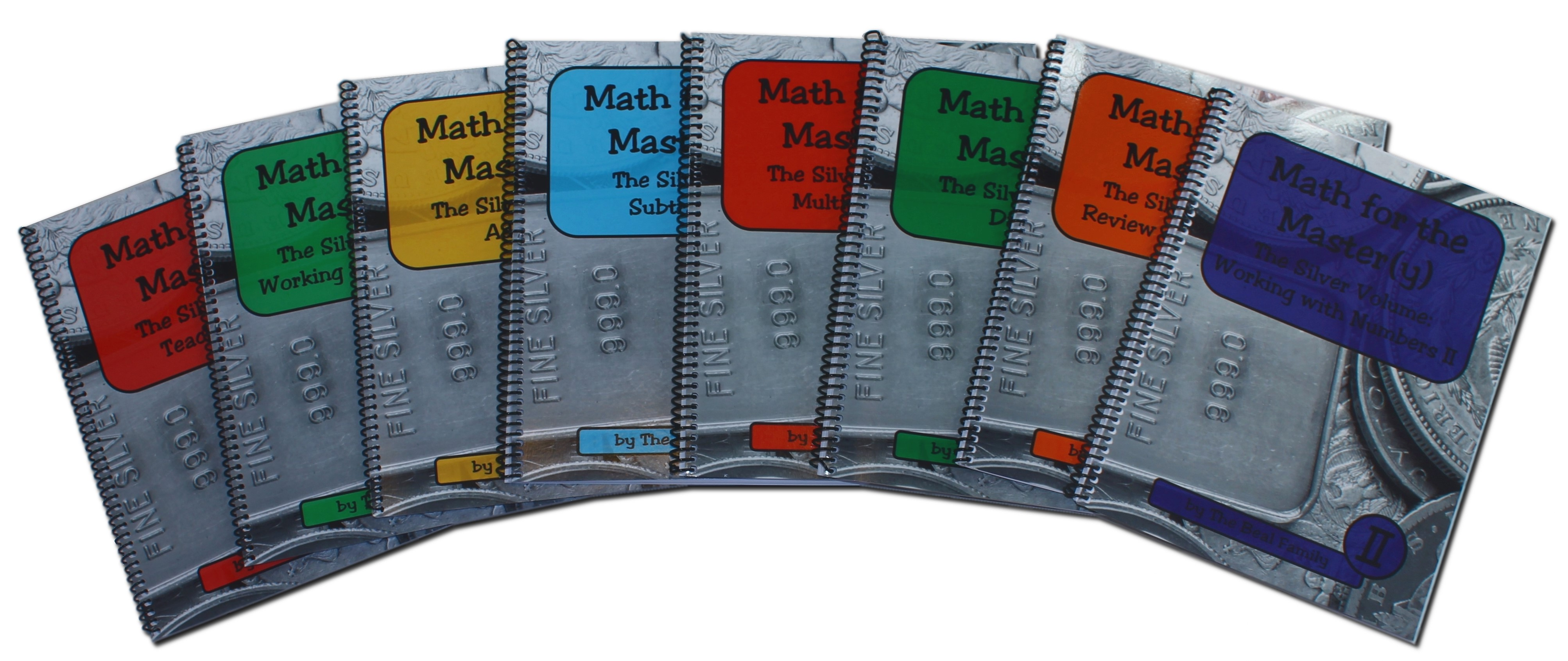Features:

• Able to be used by multiple children in a family
• Short lessons, integrates easily with daily life
• Clear directions and examples written to the child
• No more than one new concept introduced per lesson
• Large print and uncluttered pages
• Family-friendly word problems
• Able to be used multiple years, a minimum of three standard school years’ worth of material since the series contains more than 550 daily lessons … An average child will take much longer to complete all the units, if used as directed, repeating many of the lessons for mastery
• Developed by a homeschooling family specifically for homeschooling families
• Covers all four basic operations (addition, subtraction, multiplication, division) as well as “Working with Numbers” units (reading and writing numbers, Roman numerals, greater than/less than, place values, rounding, averaging, graphs, and more!)
• Recommended for ages 6 and up

Principles Underlying Creation of Math for the Master(y) (PDF)

INDIVIDUAL UNITS NOW AVAILABLE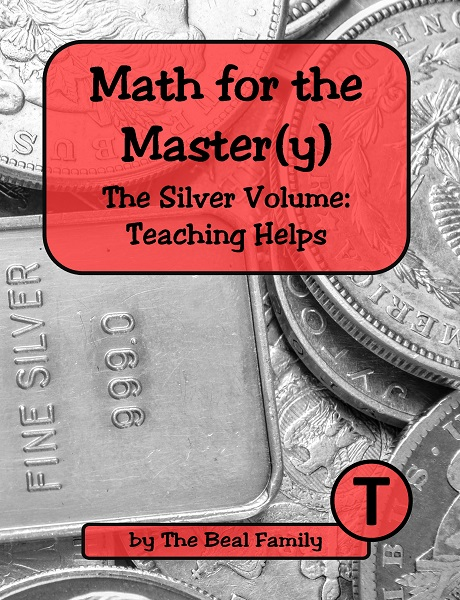Teaching Helps includes suggestions for success; FAQ; poster pages on comparing, telling time, measuring, counting money, solving word problems, and more; progress chart and certificates; topical index for all units. 8.5″ x 11″, spiral bound, 74 pages. \$14.00Sample Pages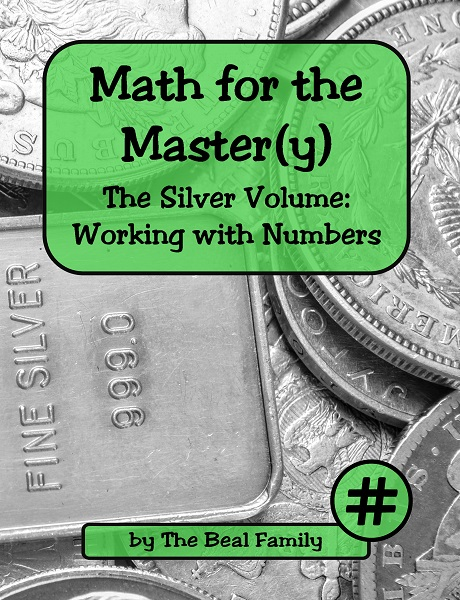Working with Numbers covers counting and writing numbers and their names (0-100); finding smallest and largest; learning less than / greater than; counting by twos, fives, tens, and hundreds; introducing place values; identifying cardinal and ordinal numbers. 8.5″ x 11″, spiral bound, 78 lessons, 85 pages. \$28.00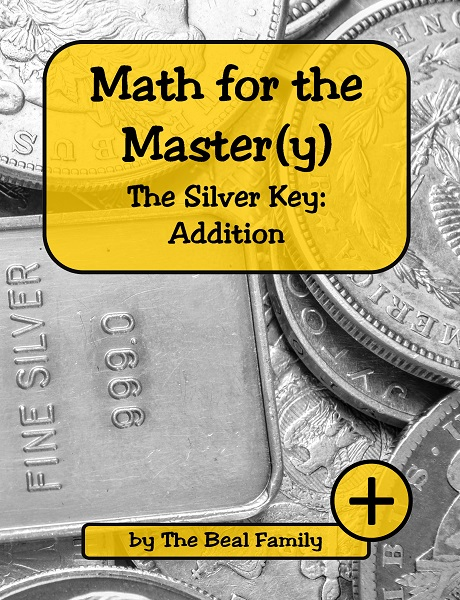Addition covers learning and reviewing addition fact families (0-9), solving word problems, adding multi-digit numbers, learning to carry, figuring mentally, checking, and using a calculator to add. 8.5″ x 11″, spiral bound, 80 lessons, 100 pages. \$30.00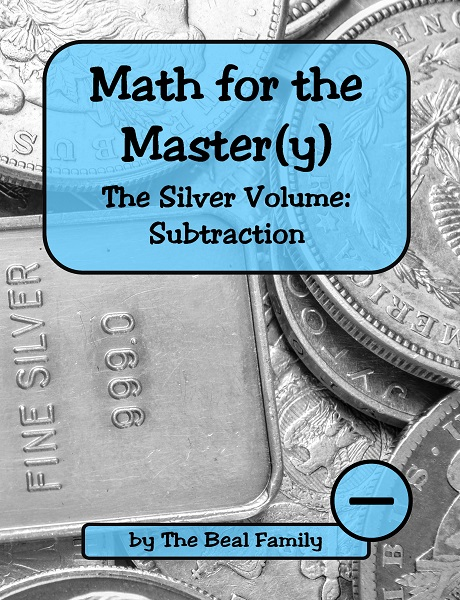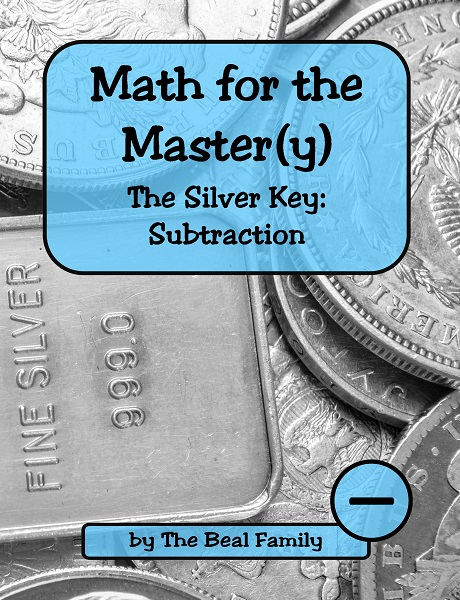Subtraction covers learning and reviewing subtraction fact families (0-9), solving word problems, subtracting multi-digit numbers, learning to borrow, figuring mentally, checking, and using a calculator to subtract. 8.5″ x 11″, spiral bound, 85 lessons, 109 pages. \$30.00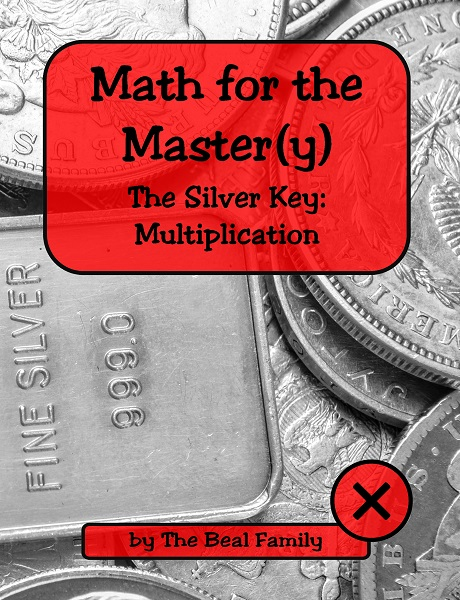Multiplication covers learning and reviewing multiplication fact families (0-12), learning to carry, multiplying larger numbers, solving word problems, multiplying by 10, 100, and 1,000, checking, and using a calculator to multiply. 8.5″ x 11″, spiral bound, 92 lessons, 120 pages. \$32.00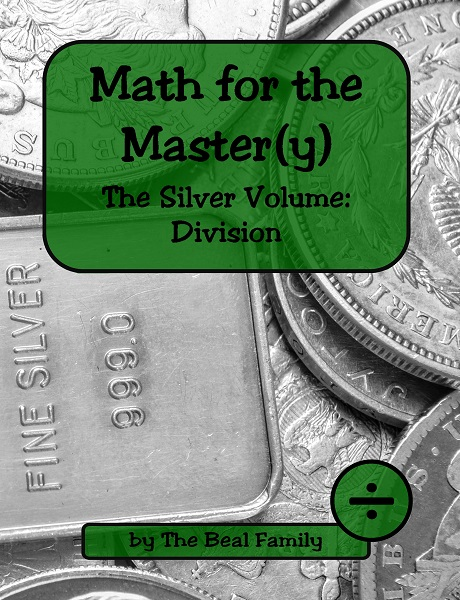Division covers learning and reviewing division fact families (0-12), dividing larger numbers, dividing with a remainder, estimating and rounding to the nearest 10, 100, and 1,000, solving word problems, checking, and using a calculator to divide. 8.5″ x 11″, spiral bound, 88 lessons, 120 pages. \$32.00.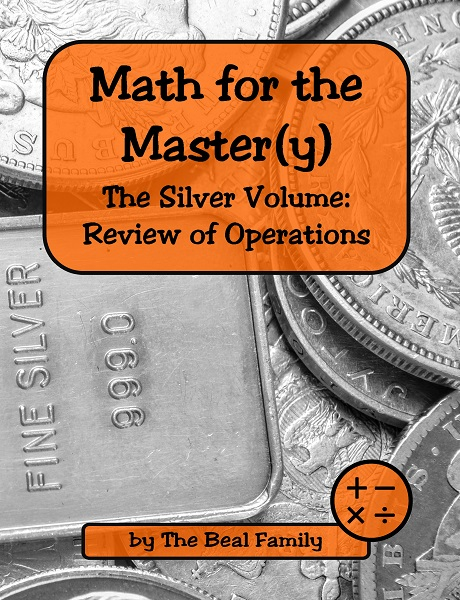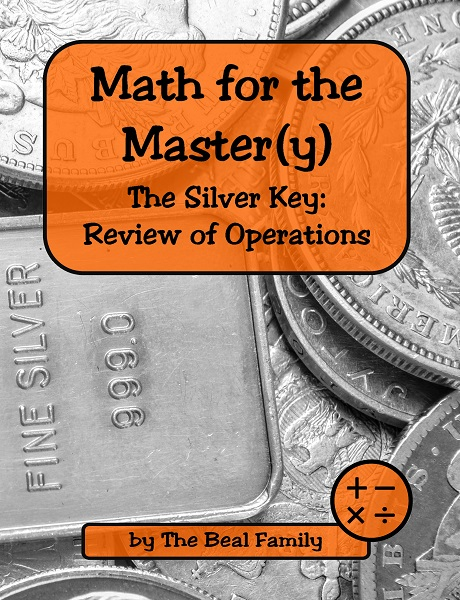Review of Operations covers recalling addition, subtraction, multiplication, and division fact families (timed), answering questions about the processes of addition, subtraction, multiplication, and division, reviewing mixed facts (timed), and solving word problems which are multi-step and/or require a variety of operations. 8.5″ x 11″, spiral bound, 50 lessons, 77 pages. \$28.00Working with Numbers II covers learning about numbers and place values for 1,000 and larger, adding and subtracting large numbers mentally, ordering large numbers, learning Roman numerals, solving problems with mixed operations, learning about mean, median, and mode, figuring with timelines, and drawing and understanding graphs. 8.5″ x 11″, spiral bound, 86 lessons, 134 pages. \$34.00

ORIGINAL HARDCOVER SILVER VOLUME
(CONTAINING ALL SEVEN UNITS LISTED ABOVE)Available at a discounted price while supplies last. We have only a few cases of the original hardcover sets remaining, and we are not planning to reorder them at this time. Selling previously for \$150. Now reduced to \$100. While some minor revisions were made between this and the newer individual units, this is still a solid product at an excellent price.

\$150 Bundle #1  Now \$100
Math for the Master(y) multi-year hardcover student volume and full-size key